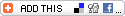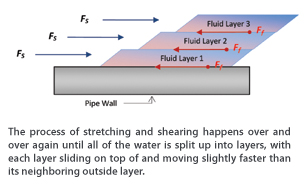Viscosity is an important property of drilling fluid because it’s the viscosity that determines the fluid’s ability to suspend and carry cuttings out of the hole. We usually think of viscosity as thickness, and we tend to approach the task of managing viscosity from our intuitive understanding of thickness.

We believe that if a drilling fluid has the right thickness, it must have the right viscosity and if it has the right viscosity, it must have good suspension characteristics and carrying capacity. Since our intuitive understanding allows us to make reasonable assessments of thickness, we believe that we can use that intuitive understanding to make reasonable assessments of viscosity, and the resulting suspension and carrying capacity. While viscosity is a way of describing thickness, it is an over-simplification to consider viscosity as the same thing as thickness as we intuitively understand it. The tools and processes that we use to manage viscosity, borne out of our intuitive understanding of thickness, may also be over-simplified, resulting in a management process that fails to effect the full work potential of the fluid.

## Water Moves In Layers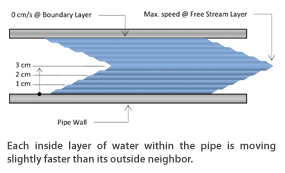To get water moving through a pipe, we have to apply a force to the water through the action of a pump. The water doesn’t simply move as a whole block, but rather as the force from the pump initiates movement. The water starts to stretch at the wall of the pipe because there is a force of friction between the pipe and the water. The friction between the water and the pipe prevents the water from simply sliding along the inside surface of the pipe. The water keeps stretching until the water breaks (or shears) at a point just above the pipe wall. The layer of water that is touching the pipe wall doesn’t move, but stays stretched out while the rest of the water moves over top of it. As the bulk of water slides over the stationary layer, a force of friction is generated between the stationary layer and the water that’s moving on top of it. This friction resists the movement of the water and causes the bulk of the water to stretch out until the water splits or shears again. This process of stretching and shearing happens over and over again until all of the water is split up into layers, with each layer sliding on top of and moving slightly faster than its neighboring outside layer.

## Shear Stress

As the layers of water slide over top of each other, friction is created between each layer. The force of the friction is transmitted through each layer, to the boundary of the water, which in this case is the wall of the pipe. The force that is exerted on the wall of the pipe is equal to the friction between each layer, all added up. If we take the total force of friction exerted against the pipe wall and divide it by the inside area of the pipe, we get the force per unit area. If we measure force in dynes and area in centimeters, we would get the force of friction in dynes/cm2. This would be the force pushing on each square centimeter of the pipe wall.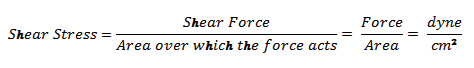Remember from our discussion above, that the water went through a process of splitting up into layers when we applied a force to it to get it moving. This force acting on each layer is called the shear force. The shear force is equal to the friction force between each layer. Since these two forces are equal, whatever we measure in terms of the force of friction is also true for the shear force. The pressure exerted against the pipe wall is not only a measure of the friction between each layer all added up, but it is also a measure of the shear force acting on each layer all added up. If we take the shear force acting on each layer, and add it all up, we get the total shear force which is pushing against the water. If we then take the total shear force and divide by the area of the pipe wall, we get the shear force acting on a 1 centimeter square column of fluid extending from the pipe wall to the center of the pipe: this is the shear stress at the pipe wall.

(1)

If we divide the shear stress at the pipe wall by the distance from the pipe wall to the center of the flow (the center of the pipe), we would get the shear stress acting on each square centimeter from the center of the flow to the pipe wall. The shear stress used in viscosity calculations is the shear stress acting on each centimeter within that column that extends from the wall to the center of flow.

## Shear Rate

Each inside layer of water within the pipe is moving slightly faster than its outside neighbor. Each layer of water increases in speed by the same amount. and the speed increase between each layer of water is the same. The water against the wall of the pipe (the boundary layer) would have a velocity of 0 cm/s, and let’s say just for example that the water in center of the pipe (the free stream layer) has a velocity of 990 cm/s. If the water is split up into 100 layers between the boundary layer and the free stream layer, then each layer would be going 9.9 cm/s faster than its outside neighbor. The incremental increase in speed is the same from layer to layer as we move from the boundary layer to the free stream layer.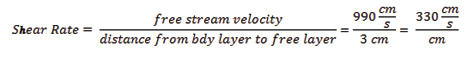The speed of each layer of water not only increases by the same amount from layer to layer, but it also increases by the same amount from centimeter to centimeter measuring radially from the boundary layer to the free stream layer. In our example, the difference in velocity, or the velocity differential, between the boundary layer and the free-stream layer is 990 cm/s. If the pipe has an internal diameter of 6 cm, then the distance between the boundary layer and the free-stream layer is 3 cm. If we divide the velocity differential of 990 cm/s by the distance between the boundary layer and the free-stream layer (3 cm) we end up with a velocity differential of 330 cm/s per centimeter. So the velocity of the water would be 0 cm/s at the boundary; 330 cm/s at 1 cm from the boundary; 660 cm/s at 2 cm from the boundary, and 990 cm/s at 3 cm from the boundary. This incremental increase in speed per centimeter is known as the shear rate. To find the shear rate, we divide the speed of the free-stream velocity layer by the distance between the boundary layer and the free-stream velocity layer.

(2)

Shear stress and shear rate are actually pretty simple concepts. There are not any more complicated than kilometers per hour.

In part two of this article, to be published in the November issue of Trenchless Technology, we will see how shear stress and shear rate are connected to viscosity and we will define exactly what viscosity is.

Manley Osbak is vice president of technical services at The Crossing Co., based in Nisku, Alberta, Canada.